# Force and Pressure Worksheet

In this page we have Force and Pressure Class 8 Worksheet . Hope you like them and do not forget to like , social share and comment at the end of the page.

## Match the Column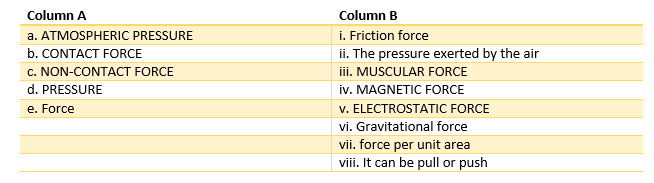## True and false

1. Friction opposes the relative motion between two surfaces in contact.
2. A force can act on an object with or without being in contact with it.
3. Gases does not exert pressure on the walls of their container
4. The force exerted by a charged body on another charged or uncharged body is known as gravitational force
5. Magnetic force is a contact force
6. Force of gravity is an attractive force
7. Atmosphere pressure at high altitudes is less than the pressure at ground
8. People at plains suffer from nose bleeding.

## Define

1. Muscular Force
2. Atmosphere pressure
3. Contact Force
4. Pressure

## Conceptual Questions

1. Why shoulder bags are provided with broad straps and not thin straps
2. Why the tools meant for cutting and piercing always have sharp edges
3. Two women are of the same weight. One wears sandals with pointed heels while the other wears sandals with flat soles. Which one would feel more comfortable while walking on a sandy beach? Give reasons for your answer.
4. Two persons are applying forces on two opposite sides of a moving cart. The cart still moves with the same speed in the same direction. What do you infer about the magnitudes and direction of the forces applied
5. Why do deep-sea divers have to wear special suits before going for diving at the depths?

## Fill in the blanks

1. An example of a non-contact force is __________.
2. The pressure exerted by air around us is known as __________.
3. SI unit of force is __________.
4. Application of force can change the ______  or  __________ of an object.
5. Force exerted by our muscles is called __________ force.
6. Direction of force of friction is always __________ to the direction of motion.
7. Force per unit area is called ___________.
8. The force exerted by a charged body on another charged or uncharged bod is known as  ______________________
9. Force has ________ as well as _________

## Multiple Choice Questions

1. A ball rolling on the ground slows down and finally stops because
(a) force
(b) less force applied
(c) friction
(d) none of the above

2. The force involved in falling of an apple from a tree is known as?
(a) magnetic force
(b) electrostatic force
(c) contact force
(d) gravitational force

3. Which of the following is an example of a non-contact force?
(a) The force exerted by us to lift a bucket
(b) push a stationary car
(c) The force exerted by magnets
(d) Force due to friction

4. The below figure shows a container filled with water. Which of the following statements is correct about pressure of water?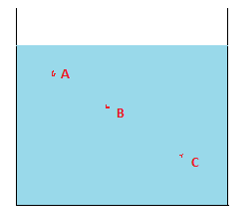(a) Pressure at A> Pressure at B> Pressure at C
(b) Pressure at A=Pressure at B=Pressure at C
(c) Pressure at A< Pressure at B>Pressure at C
(d) Pressure at A< Pressure at B < Pressure at C

5. Which one of the following forces is a contact force?
(a) force of gravity
(b) magnetic force
(c) force of friction
(d) electrostatic force

6.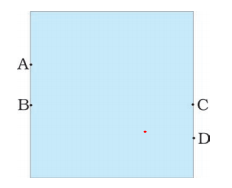A water tank has four taps fixed at points A, B, C, D as shown above
The water will flow out at the same pressure from taps at
(a) B and C
(b) C and D
(c) A and B
(d) A and C

7. A brick is kept in two different ways on a table as shown below. The pressure exerted by the brick on the table will be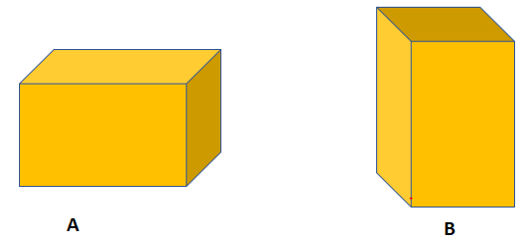(a) maximum in position A
(b) maximum in position B
(c) equal in both cases

8. Opening the door is an example of which type of force?
(a) contact force
(b) spring force
(c) non-contact force
(d) magnetic force

9. Name the force used in removing iron scrap from a heap of mixed scrap.
(a)  Magnetic Force
(b) Electrostatic force
(c) Gravitational force
(d) Friction Force

10.  The pressure on an object _______ with the increase in altitude  from earth surface
(a) Increase
(b) decrease
(c) same
(d) None

## JUMBLED WORD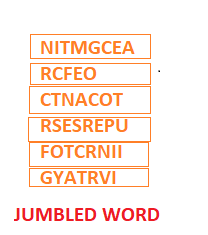## Crossword Puzzle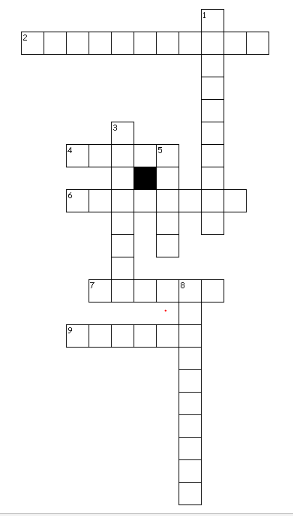Across
2. The pressure exerted by air around us is known as ______ pressure
4. Push or pull on an object is known as _____
6. The force resulting due to the action of muscles is known as the  ________force
7. A _____ scientist of 17th century, invented a pump to extract air out of the vessel
9. Pressure exerted by water at the bottom of the container depends on the _______ of its column
Down
1. ______ force always acts opposite to the motion of the object
3. Force per unit area
5. Liquids exert ______ pressure at the same depth
8. gravity is an _____ force

## Summary

This Force and Pressure Worksheet for Class 8 is prepared keeping in mind the latest syllabus of CBSE . This has been designed in a way to improve the academic performance of the students. If you find mistakes , please do provide the feedback on the mail.